# NthDigit

Contributed by: Paco Jain (Wolfram Research)

Computes the digit in a given place of the positional representation of a number

 ResourceFunction["NthDigit"][x,n] Returns the nth base-10 digit of x. ResourceFunction["NthDigit"][x,n,base] Returns the nth digit of x in the specified base.

## Details and Options

ResourceFunction["NthDigit"][x,n] returns the nth most significant digit of x when expressed in standard positional form, padding with zeros to the right of the decimal if necessary.
For numbers x<1, ResourceFunction["NthDigit"][x, 1] gives 0 and ResourceFunction["NthDigit"][x, n] gives the digit n-1 places to the right of the decimal point.
If n is larger than Precision[x]/Log[10, b], then ResourceFunction["NthDigit"][x,n,b] returns Indeterminate.
The base b in ResourceFunction["NthDigit"][x,n,b] need not be an integer. For any real b such that b > 1, ResourceFunction["NthDigit"][x,n,b] finds the largest integer multiples of bn that can be subtracted from x while leaving a non-negative remainder.
ResourceFunction["NthDigit"][x,n,b] discards the sign of b.

## Examples

### Basic Examples

Compute the nth digit of a given number:

 In:=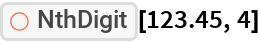Out=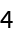Give the 2500th digit of π in base-10:

 In:=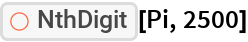Out=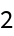Compute the base-10 digits of a number less than 1:

 In:=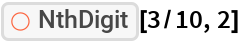Out=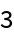In:=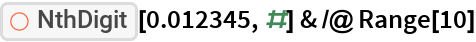Out=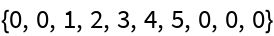Give the first 10 digit of 19/7 in base 3:

 In:=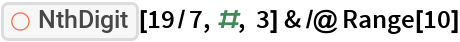Out=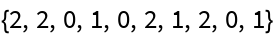### Scope

Noninteger bases are allowed:

 In:=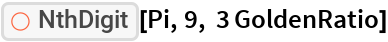Out=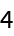### Applications

Compute the number of 1s in the first 10^4 binary digits of π:

 In:=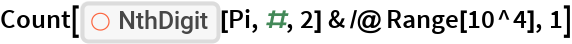Out=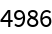Plot the distribution of first 10,000 digits of π in base-47:

 In:=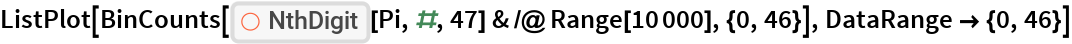Out=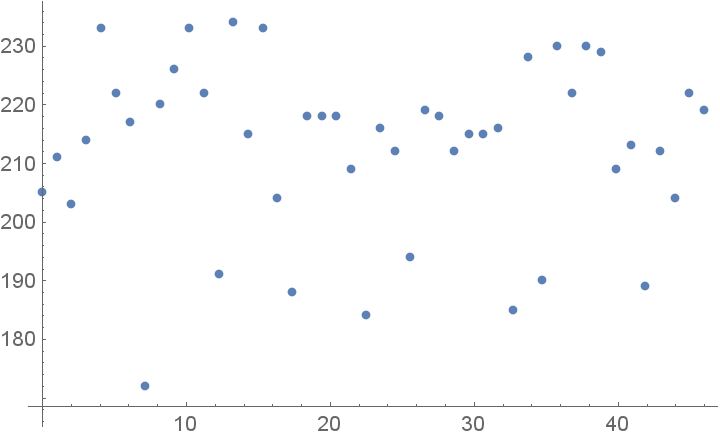### Properties and Relations

NthDigit ignores the sign of its first argument:

 In:=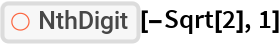Out=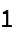NthDigit gives Indeterminate if more digits than the precision are requested:

 In:=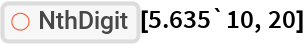Out=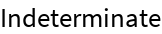In:=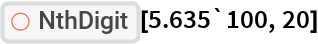Out=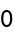### Possible Issues

Positional representations only work for bases greater than 1. Using an unsuitable base triggers a message:

 In:=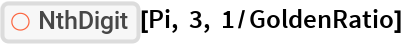Out=